Electron. J. Diff. Eqns., Vol. 2002(2002), No. 53, pp. 1-17.

### Uniqueness theorem for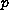-biharmonic equations Jiri Benedikt

Abstract:
The goal of this paper is to prove existence and uniqueness of a solution of the initial value problem for the equation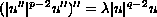where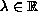and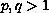. We prove the existence for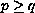only, and give a counterexample which shows that for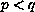there need not exist a global solution (blow-up of the solution can occur). On the other hand, we prove the uniqueness for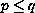, and show that for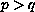the uniqueness does not hold true (we give a corresponding counterexample again). Moreover, we deal with continuous dependence of the solution on the initial conditions and parameters.

An addendum was attached on July 28, 2003. In Remark 4.6, fourth line (page 15) there should be $u(t)=K(t-t_0)^r$ instead of $u(t)=K(H-t)^r$.

Submitted April 15, 2002. Published June 10, 2002.
Math Subject Classifications: 34A12, 34C11, 34L30.
Key Words: p-biharmonic operator, existence and uniqueness of solution, continuous dependence on initial conditions, jumping nonlinearity.

Show me the PDF file (303K), TEX file, and other files for this article.Jiri Benedikt Centre of Applied Mathematics University of West Bohemia Univerzitni 22, 306 14 Plzen Czech Republic e-mail: benedikt@kma.zcu.cz